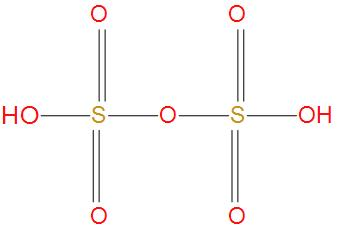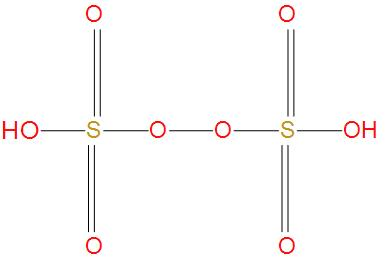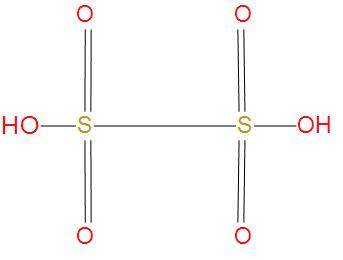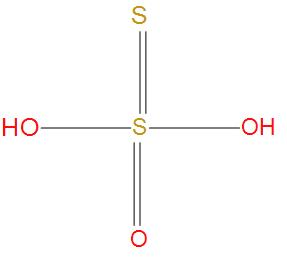QuestionAnswers

# Which oxyacid of sulphur contains S-S single bond?A. OleumB. Marshall’s acidC. Dithionic acidD. Thiosulphuric acid

Hint: Oxyacid is a compound that contains oxygen, hydrogen and one other element. Here, we are provided with oxoacids of sulphur, these contain oxygen, hydrogen and sulphur atoms.

- Oleum is also called as pyrosulfuric acid. The molecular formula of oleum is ${{H}_{2}}{{S}_{2}}{{O}_{7}}$. We can see the structure of oleum:- We can see from the structure that there is no S-S single bond present, hence this option is wrong.
- Next option is Marshall’s acid, we also called it as peroxydisulfuric acid, its molecular formula is ${{H}_{2}}{{S}_{2}}{{O}_{8}}$ . We will see the structure of this:- Here, we can see that there is one peroxy linkage that O-O is present, and there is no S-S single bond present. Hence, this option is wrong.
- Let’s see the next option Dithionic acid, its molecular formula is ${{H}_{2}}{{S}_{2}}{{O}_{6}}$. We will see the structure of this:- We can see from the structure that there is one S-S single bond present. And we can say that this option is correct.
- Similarly, in Thiosulphuric acid, there is no S-S single bond present. We can see this from structure:Hence, we can conclude that the correct option is (C), Dithionic acid contains S-S single bond.

Note: We know that an oxyacid molecule contains the structure X-O-H, where O is oxygen, H is hydrogen and X is any element. Where, other atoms can be connected to the central metal atom X. In a solution, oxyacid can be dissociated as: $X-O-H\rightleftarrows {{\left( X-O \right)}^{-}}+H$